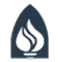## GPS Time Conversion AlgorithmThe GPS Time Converter perfoms conversions between GPS and "human" time. There are many programs and functions available (including the built in php functions used by the converter) for converting between "human" time and Unix time. The more difficult part of the conversion is going between Unix time and GPS time. This is because while there was an offset of 315964800 seconds between Unix and GPS time when GPS time began, that offset changes each time there is a leap second. GPS time labels each second uniquely including leap seconds while Unix time does not, preferring to count a constant number of seconds a day including those containing leap seconds.

Below are the PHP functions used to do the conversion between GPS time and Unix time. Note that for leap seconds the Unix time is given an additional .5 seconds from the previous second to distinguish it from the seconds immediately before and afterwards. In addition, a javascript GPS time converter written by Jeff Kline and Roy Williams is available here.

``` // Define GPS leap seconds function getleaps() { \$leaps = array(46828800, 78364801, 109900802, 173059203, 252028804, 315187205, 346723206, 393984007, 425520008, 457056009, 504489610, 551750411, 599184012, 820108813, 914803214, 1025136015, 1119744016, 1167264017); return \$leaps; } // Test to see if a GPS second is a leap second function isleap(\$gpsTime) { \$isLeap = FALSE; \$leaps = getleaps(); \$lenLeaps = count(\$leaps); for (\$i = 0; \$i < \$lenLeaps; \$i++) { if (\$gpsTime == \$leaps[\$i]) { \$isLeap = TRUE; } } return \$isLeap; } // Count number of leap seconds that have passed function countleaps(\$gpsTime, \$dirFlag){ \$leaps = getleaps(); \$lenLeaps = count(\$leaps); \$nleaps = 0; // number of leap seconds prior to gpsTime for (\$i = 0; \$i < \$lenLeaps; \$i++) { if (!strcmp('unix2gps', \$dirFlag)) { if (\$gpsTime >= \$leaps[\$i] - \$i) { \$nleaps++; } } elseif (!strcmp('gps2unix', \$dirFlag)) { if (\$gpsTime >= \$leaps[\$i]) { \$nleaps++; } } else { echo "ERROR Invalid Flag!"; } } return \$nleaps; } // Convert Unix Time to GPS Time function unix2gps(\$unixTime){ // Add offset in seconds if (fmod(\$unixTime, 1) != 0) { \$unixTime = \$unixTime - 0.5; \$isLeap = 1; } else { \$isLeap = 0; } \$gpsTime = \$unixTime - 315964800; \$nleaps = countleaps(\$gpsTime, 'unix2gps'); \$gpsTime = \$gpsTime + \$nleaps + \$isLeap; return \$gpsTime; } // Convert GPS Time to Unix Time function gps2unix(\$gpsTime){ // Add offset in seconds \$unixTime = \$gpsTime + 315964800; \$nleaps = countleaps(\$gpsTime, 'gps2unix'); \$unixTime = \$unixTime - \$nleaps; if (isleap(\$gpsTime)) { \$unixTime = \$unixTime + 0.5; } return \$unixTime; } ```

If you have any questions or suggestions regarding this page, please send them to tzs at andrews dot edu.